# RD Sharma Solutions Class 7 Chapter 17 Constructions

Read RD Sharma Solutions Class 7 Chapter 17 Constructions below, students should study RD Sharma class 7 Mathematics available on Studiestoday.com with solved questions and answers. These chapter wise answers for class 7 Mathematics have been prepared by teacher of Grade 7. These RD Sharma class 7 Solutions have been designed as per the latest NCERT syllabus for class 7 and if practiced thoroughly can help you to score good marks in standard 7 Mathematics class tests and examinations

Exercise 17.1

Question 1: Draw an ∠BAC of measure 50o such that AB = 5 cm and AC = 7 cm. Through C draw a line parallel to AB and through B draw a line parallel to AC, intersecting each other at D. Measure BD and CD

Solution 1: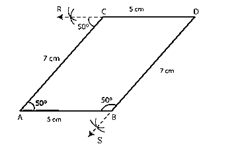Construction:

Step 1. Make an angle BAC = 50o such that AB = 5 cm and AC = 7 cm. and cut an arc through point C at an angle 50o.
Step 2. Make a straight line through point C and the arc. This line will be parallel to AB, ∠CAB =∠RCA=50o
Step 3. Alternate angles are equal; thus, the line is parallel to AB.
Step 4. Now, through point B, cut an arc at an angle of 50o and Make a line passing through B and this arc and say this intersects the line drawn parallel to AB at D.
Step 5. ∠SBA =∠BAC = 50o, since they are alternate angles. Thus, BD parallel to AC
Step 6. And also, we measure BD = 7 cm and CD = 5 cm.

Question :2. Draw a line PQ.  Draw another line parallel to PQ at a distance of 3 cm from it.

Solution  2: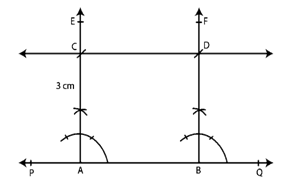Construction:
Step 1. Make a line PQ.
Step 2. Select any two points on the line and name them A and B.
Step 3. Construct ∠PBF = 90o and ∠QAE = 90o
Step 4. Use point A as center and radius 3 cm cut AE at C.
Step 5. Use point B as center and radius 3 cm cut BF at D.
Step 6. Connect point CD and produce it on either side to get the required line parallel to AB and at a distance of 3 cm from it.

Question :3. Take any three non-collinear points A, B, C and draw ∠ABC. Through each vertex of the triangle, draw a line parallel to the opposite side.

Solution  3: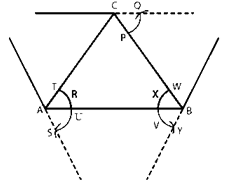Construction:

Step 1. Mark A, B and C three non collinear points such that none of them lie on the same line.
Step 2. Form a triangle ABC, by joining AB, BC, and CA.
Step 3. Parallel line to AC.
Step 4. Use point A as center, make an arc cutting AC and AB at T and U, respectively.
Step 5. Use center point B and at the same radius as in the previous step, make an arc on the opposite side of AB to cut AB at X.
Step 6. Use center X and radius equal to TU, Make an arc cutting the arc drawn in the previous step at Y.
Step 7. Connect BY and produce in both directions to obtain the line parallel to AC.
Parallel line to AB:
Step 8. Use point B as center, Make an arc cutting BC and BA at W and V, respectively.
Step 9. Use center C and the same radius as in the previous step, make an arc on the opposite side of BC to cut BC at P.
Step 10. Create an arc cutting the arc and making in the previous phase at Q using centre P and radius equal to WV.
Step 11. Make a line parallel to AB by connecting CQ and producing in both directions.
Parallel line to BC:
Step 12. Make an arc with B as the middle, cutting BC and BA at W and V, respectively.
Step 13. Make an arc on the opposite side of AB to cut AB at R, using centre A and the same radius as in the previous step.
Step 14. Make an arc with a radius equal to WV and a center R that intersects the arc drawn in the previous phase at S.
Step 15. Connect AS and produce in both directions to obtain the line parallel to BC.

Question :4. Draw two parallel lines at a distance of 5cm apart.

Solution  4:Construction:

Step 1. Make a line PQ.
Step 2. Select any two points A and B on the line PQ.
Step 3. Construct ∠PBF = 90o and ∠QAE = 90o
Step 4. Use point A as center and radius 5 cm cut AE at C.
Step 5. Use point B as center and radius 5 cm cut BF at D.
Step 6. Connect CD and make the appropriate line parallel to AB and at a distance of 5 cm from it on either side.

Exercise 17.2

Question :1. Draw △ABC in which AB = 5.5 cm. BC = 6 cm and CA = 7 cm. Also, draw perpendicular bisector of side BC.

Solution 1: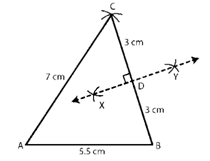Construction:
Step 1. Make a line segment AB of length 5.5 cm.
Step 2. Cut a 6 cm radius arc from B.
Step 3. Make an arc with a radius of 7 cm from center A, intersecting the previously drawn arc at C.
Step 4. To make the desired triangle, link AC and BC.
Step 5. Make two arcs on both sides of BC, with center B and a radius greater than half of BC.
Step 6. Make two arcs intersecting the arcs drawn in the previous step at X and Y with center C and the same radius as in the previous step.
Step 7. Connect XY to obtain BC's perpendicular bisector.

Question :2. Draw ∆PQR in which PQ = 3 cm, QR = 4 cm and RP = 5 cm. Also, draw the bisector of ∠Q

Solution 2: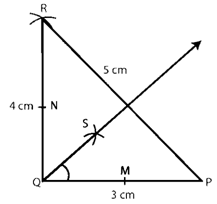Construction:
Step 1. Make a line segment PQ of length 3 cm.
Step 2. Make an arc with Q as the core and a radius of 4 cm.
Step 3. Make an arc intersecting the previously drawn arc at R with P as the middle and a radius of 5 cm.
Step 4. To make the appropriate triangle, connect PR and QR.
Step 5. Cut equal-radius arcs from Q, intersecting PQ and QR at M and N, respectively.
Step 6. Cut arcs of equal radius from M and N, intersecting at point S.
Step 7. Establish the angle bisector of angle PQR by joining QS and extending it.
Step 8. Check that the angles PQS and SQR are both 45o.

Question :3. Draw an equilateral triangle one of whose sides is of length 7 cm.

Solution  3: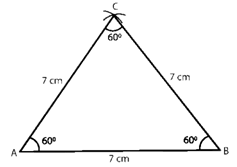Construction:
Step 1. Make a line segment AB with length 7 cm.
Step 2. Use center point A, make an arc of radius 7 cm.
Step 3. Use center point B, make an arc of radius 7 cm intersecting the previously drawn arc at C.
Step 4. Connect AC and BC to get the required triangle.

Question :4. Draw a triangle whose sides are of lengths 4 cm, 5 cm and 7 cm. Draw the perpendicular bisector of the largest side.

Solution  4:Construction:
Step 1. Make a 7-cm-long line segment PR.
Step 2. Make a 5 cm radius arc with center P.
Step 3. Make an arc of radius 4 cm with center R intersecting the previously drawn arc at Q.
Step 4. To make the appropriate triangle, connect PQ and QR.
Step 5. On either side of P, Make arcs with a radius greater than half of PR.
Step 6. Make arcs from R on both sides of PR, intersecting the arcs drawn in the previous step at M and N, with the same radius as in the previous step.
Step 7. MN is the perpendicular bisector of the largest side that is needed.

Question :5. Draw a triangle ABC with AB = 6 cm, BC = 7 cm and CA = 8 cm. Using ruler and compass alone, draw (i) the bisector AD of ∠A and (ii) perpendicular AL from A on BC. Measure LAD.

Solution  5: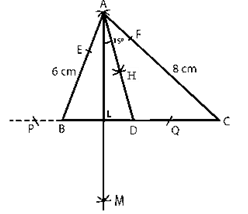Construction:
Step 1. Make a 7-cm-long line segment BC.
Step 2. Make a 6 cm radius arc with center B.
Step 3. Make an arc of radius 8 cm with center C intersecting the previously drawn arc at A.
Step 4. To make the requisite triangle, connect AC and AB.
Angle bisector steps:
Step 5. Cut equal-radius arcs from A, intersecting AB and AC at E and F, respectively.
Step 6. Cut arcs of equal radius from E and F, intersecting at point H.
Step 7. Connect AH and extend it to form the angle bisector of angle A, which intersects line BC at D.
Step 8. Steps perpendicular to the line BC from Point A.
Step 9. Cut arcs of equal radius from A that intersect BC at P and Q, respectively (Extend BC to Make these arcs).
Step 10. Cut arcs of equal radius from P and Q, intersecting at M.
Step 11. Cut BC at L with AM.
Step 12. The line BC is perpendicular to AL.
Step 13. LAD has a 15o angle.

Question :6. Draw △DEF such that DE= DF= 4 cm and EF = 6 cm. Measure ∠E and ∠F.

Solution  6:Construction:
Step 1. Make a 6 cm long EF line segment.
Step 2. Make an arc with a radius of 4 cm with E as the middle.
Step 3. Make an arc of radius 4 cm with F as the origin, intersecting the previous arc at D.
Step 4. Link DE and DF to form the DEF triangle.
Step 5. We get ∠E= ∠F= 40o by measuring.

Question :7. Draw any triangle ABC. Bisect side AB at D. Through D, draw a line parallel to BC, meeting AC in E. Measure AE and EC.

Solution  7:Firstly, Make a triangle, Name then ABC with each side = 6 cm.
Construction to bisect line AB:
Step 1. On either side of line AB, Make an arc from A.
Step 2. Make an arc from B on either side of AB, intersecting the arcs drawn in the previous step at P and Q, with the same radius as in the previous step.
Step 3. Connect PQ at D, cutting AB. The perpendicular bisector of AB is PQ.
Parallel line to BC:
Step 4. Make an arc with B as the base, cutting BC and BA at M and N, respectively.
Step 5. Make an arc on the opposite side of AB to cut AB at Y using center D and the same radius as in the previous step.
Step 6. With a radius of MN and a center of Y, Make an arc that intersects the arc drawn in the previous phase at X.
Step 7. Connect XD and extend it to E, where it intersects AC.
Step 8. The requisite parallel line is DE.

Exercise 17.3

Question :1. Draw △ABC in which AB = 3 cm, BC = 5 cm and ∠B = 70o.

Solution  1:Construction:
Step 1. Make a 3 cm long line segment AB.
Step 2. Make the angle ∠XBA=70o.
Step 3. At C, cut an arc on BX at a 5 cm radius.
Step 4. Connect AC to complete the triangle.

Question :2. Draw △ABC in which ∠A=70o. AB = 4 cm and AC= 6 cm. Measure BC.

Solution  2:Construction:
Step 1. Make a 6 cm long line segment AC.
Step 2. Make an angle ∠XAC=70o.
Step 3. At B, Make an arc on AX at a distance of 4 cm.
Step 4. Connect BC to form the triangle you want.
Step 5. We can see that BC is equal to 6 cm.

Question :3. Draw an isosceles triangle in which each of the equal sides is of length 3 cm and the angle between them is 45o.

Solution  3:Construction:
Step 1. Make a 3 cm long PQ line segment.
Step 2. Make a ∠QPX=45o drawing.
Step 3. On PX, cut an arc at R at a distance of 3 cm.
Step 4. Connect QR to get the triangle.

Question :4. Draw △ABC in which ∠A = 120o, AB = AC = 3 cm. Measure ∠B and ∠C.

Solution  4: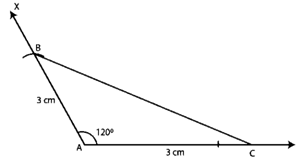Construction:
Step 1. Make a 3 cm long line segment AC.
Step 2. Make the angle ∠XAC = 120o.
Step 3. At B, Make an arc on AX at a distance of 3 cm.
Step 4. Connect BC to complete the triangle.
Step 5. We get ∠B = ∠C = 30o by measuring.

Question :5. Draw △ABC in which ∠C = 90o and AC = BC = 4 cm.

Solution  5:Construction:
Step 1. Make a 4 cm long line segment BC.
Step 2. Make ∠BCY=90° at C.
Step 3. At A, Make an arc on CY at a distance of 4 cm.
Step 4. connect AB, ABC is the required triangle.

Question :6. Draw a triangle ABC in which BC = 4 cm, AB = 3 cm and ∠B = 45o. Also, draw a perpendicular from A on BC.

Solution  6: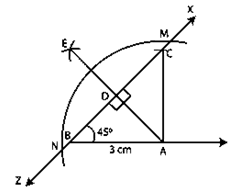Construction:
Step 1. Make a 3 cm long line segment AB.
Step 2. At C, Make a 45o angle and cut an arc with a radius of 4 cm at this angle.
Step 3. Connect AC to complete the triangle.
Step 4. Make intersecting arcs at M and N with A as the middle.
Step 5. Cut an arc on the opposite side of A with a middle M and a radius greater than half of MN.
Step 6. Cut an arc intersecting the previous arc at E with N as the center and the same radius as in the previous stage.
Step 7. Connect AE at D, where it meets BC, and AE becomes the appropriate perpendicular.

Question :7. Draw a triangle ABC with AB = 3 cm, BC = 4 cm and ∠B = 60o. Also, draw the bisector of angles C and A of the triangle, meeting in a point O. Measure ∠COA.

Solution  7: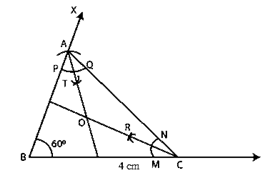Construction:
Step 1. Make a BC = 4 cm line segment.
Step 2. Make an angle ∠CBX = 60o.
Step 3. Make a 3 cm radius arc on BX, cutting BX at A.
Step 4. Connect AC to complete the triangle.
Angle bisector for angle A:
Step 5. Cut arcs of the same radius with A as the middle, cutting AB and AC at P and Q, respectively.
Step 6. Cut arcs of the same radius from P and Q, intersecting at T.
Step 7. Combine AT and A to get the angle bisector of A.
Angle bisector for angle C:
Step 8.Cut arcs of the same radius with A as the middle, cutting CB and CA at M and N, respectively.
Step 9. Cut arcs of the same radius intersecting at R from M and N.
Step 10. To get the angle bisector of angle C, connect CR.
Step 11. Make an O at the intersection of CR and AT.
Step 12. Angle ∠COA = 120o

Exercise 17.4

Question :1. Construct ∆ABC in which BC = 4 cm, ∠B = 50o and ∠C = 70o.

Solution  1: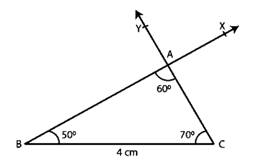Construction:
Step 1. Make a 4 cm long line segment BC.
Step 2. Make ∠CBX, so that it equals ∠CBX=50o.
Step 3. Make ∠BCY =70o by placing Y on the same side of BC as X.
Step 4. Assume that CY and BX cross at A.
Step 5. The requisite triangle is ABC.

Question :2. Draw ∆ABC in which BC = 8 cm, ∠B = 50o and ∠A = 50o.

Solution  2: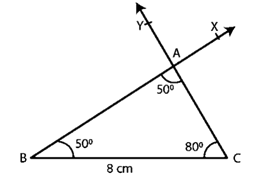Construction:
Step 1. Make an 8-cm-long line segment BC.
Step 2. Make ∠CBX so that it equals ∠CBX = 50o.
Step 3. Make ∠BCY so that ∠BCY = 80o, with Y on the same side of BC as X.
Step 4. Assume that CY and BX cross at A.

Question :3. Draw ∆ABC in which ∠Q = 80o, ∠R = 55o and QR = 4.5 cm. Draw the perpendicular bisector of side QR.

Solution  3:Construction:
Step 1. Make a QR = 4.5 cm line section.
Step 2. Make ∠RQX = 80° and ∠QRY = 55o.
Step 3. Assume that QX and RY intersect at P, forming the appropriate triangle PQR.
Step 4. Make arcs on both sides of QR with Q as the middle and a radius greater than 4.5 cm.
Step 5. Make arcs intersecting the previous arcs at M and N with R as the middle and a radius greater than 4.5 cm.
Step 6. Connect MN.
Step 7. MN is the perpendicular bisector of QR that is necessary.

Question :4. Construct ∆ABC in which AB = 6.4 cm, ∠A = 45o and ∠B = 60o

Solution  4:Construction:
Step 1. Make a line segment with the length AB = 6.4 cm.
Step 2. Make ∠BAX = 45o angle.
Step 3. ∠ABY should be drawn with Y on the same side of AB as X, so that ∠ABY = 60o
Step 4. Assume the AX and BY cross at C.
Step 5. The requisite triangle is ABC.

Question :5. Draw ∆ABC in which AC = 6 cm, ∠A = 90o and ∠B = 60o

Solution 5: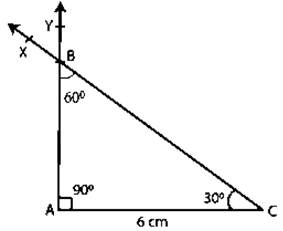Construction:
Step 1. Make a line segment with the length AC = 6 cm.
Step 2. Make ∠ACX = 30o angle.
Step 3. Make ∠CAY so that Y is on the same side of AC as X and ∠CAY = 90o.
Step 4. Team up with CX and AY. Allow these to cross at B.
Step 5. The required triangle is ABC, with an angle of ∠ABC = 60o

Exercise 17.5

Question :1. Draw a right triangle with hypotenuse of length 5 cm and one side of length 4 cm.

Solution  1: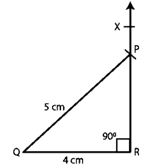Construction:
Step 1. Make a QR = 4 cm line section.
Step 2. Make an angle 90o ∠QRX.
Step 3. Make an arc of the triangle with a middle Q and a radius PQ = 5 cm to intersect ray RX at P.
Step 4. Combine PQ and PQR to form the ideal triangle PQR.
Step 5. The required triangle is PQR.

Question :2. Draw a right triangle whose hypotenuse is of length 4 cm and one side is of length 2.5 cm.

Solution 2:Construction:
Step 1. Make a 2.5-cm-long line segment QR.
Step 2. Make a 90o ∠QRX.
Step 3. Make an arc of the triangle with a middle Q and a radius PQ = 4 cm to intersect ray RX at P.
Step 4. Combine PQ and PQR to form the ideal triangle PQR.
Step 5. The required triangle is PQR.

Question :3. Draw a right triangle having hypotenuse of length 5.4 cm, and one of the acute angles of measure 30o

Solution  3:Let ABC be the right triangle at A such that hypotenuse BC = 5.4 cm. Let C = 30o.
Therefore ∠A + ∠B + ∠C = 180o
∠B = 180o − 30o− 90o = 60o
Construction:
Step 1. Make a BC = 5.4 cm line section.
Step 2. Make a 60o angle CBY.
Step 3. Make a 30o angle BCX on the same side of BC as Y, with X on the same side of BC as Y.
Step 4. Assume that BY and CX cross at A.
Step 5. The requisite triangle is then ABC.

Question :4. Construct a right triangle ABC in which AB = 5.8 cm, BC = 4.5 cm and ∠C = 90o.

Solution  4: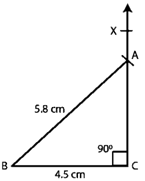Construction:
Step 1. Make a BC = 4.5 cm line section.
Step 2. Make a BCX with a 90 o angle.
Step 3. Make an arc of the triangle to intersect ray CX at A, with center B and radius AB = 5.8 cm
Step 4. Connect AB to make the triangle ABC you want.
Step 5. The requisite triangle is ABC.

Question :5. Construct a right triangle, right angled at C in which AB = 5.2 cm and BC= 4.6 cm.

Solution  5: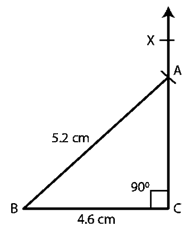Construction:
Step 1. Make a BC = 4.6 cm line section.
Step 2. Make ∠BCX of measure 90o
Step 3. Make an arc of the triangle with a center B and a radius AB = 5.2 cm that intersects ray CX at A.
Step 4. To get the desired triangle ABC, connect AB.
Step 5. The requisite triangle is ABC.